output.to from Sideway
Draft for Information Only

# Content

`Vector Components Components of Vector  One Dimension  Two Dimension  Three Dimension Vector in Space`

# Vector Components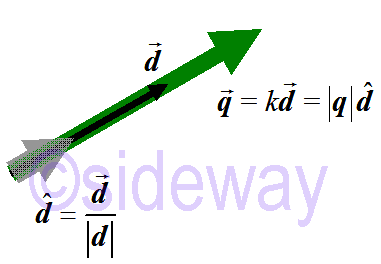A vector is used to represent the magnitude and direction of a vector quantity. A vector quantity, 𝑄, is therefore can be expressed as a simple vector 𝒒. Or in form of a scaled vector by simple geometry operation, that is a scalar 𝑘 times a directional vector 𝒅 or the magnitude |𝒒| times a unit directional vector 𝒅. In other words, the study of vector components can only focus on the directional vector irrespective of its magnitude.

## Components of Vector

In general, the values that are used to represent a vector are called the components of the vector, and the number of components used to define a vector is equal to the number of dimensions of interest.

### One Dimension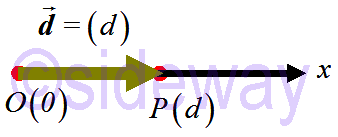Since a one-dimensional vector always lies along a line, only one value is needed to specify the vector. There is only one component for a one-dimensional vector. For example, 𝒅=(𝑑)

### Two Dimension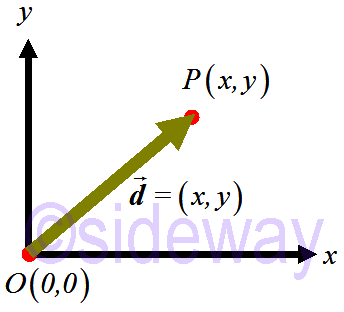Since a two-dimensional vector always lies in a Cartesian plane, two values are needed to specify the vector. There are two components for a two-dimensional vector. For example, 𝒅=(𝑥,𝑦).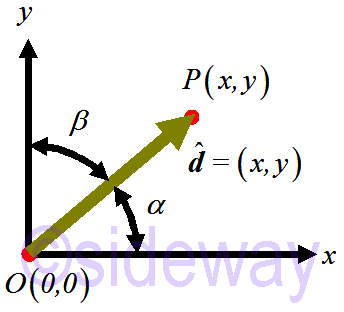If 𝒅 is a unit vector, the components of the vector can be expressed in terms of angles between the vector and coordinate axes, that is 𝑥=cos 𝛼 and 𝑦=cos 𝛽. Therefore 𝒅=(cos 𝛼,cos 𝛽)
Since the components of a vector is an ordered set, the rectangular Cartesian coordinate system of components 𝑥 and 𝑦 must be arranged in correct order following the right-hand rule.

### Three Dimension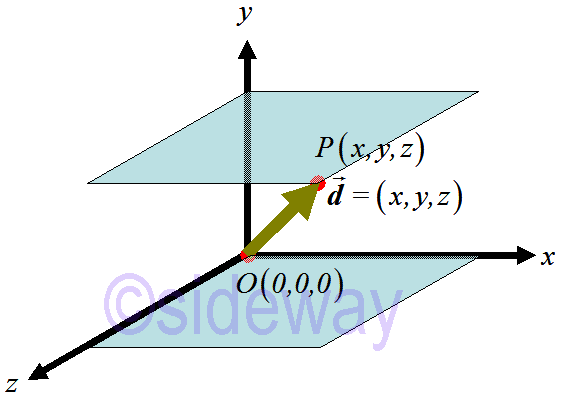Since a three-dimensional vector always lies in a Cartesian space, three values are needed to specify the vector. There are three components for a three-dimensional vector. For example, 𝒅=(𝑥,𝑦,𝑧).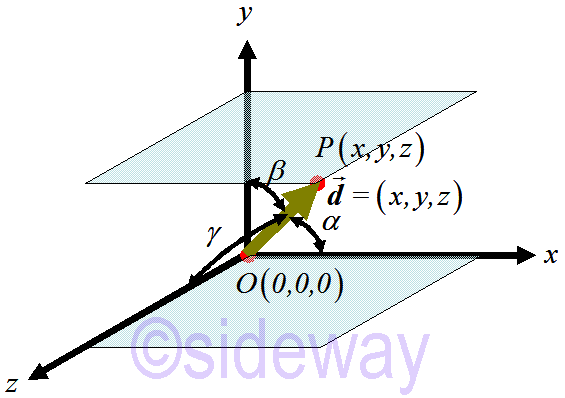If 𝒅 is a unit vector, the components of the vector can be expressed in terms of angles between the vector and coordinate axes, that is 𝑥=cos 𝛼, 𝑦=cos 𝛽, and 𝑧=cos 𝛾. Therefore 𝒅=(cos 𝛼,cos 𝛽,cos 𝛾)
Since the components of a vector is an ordered set, the rectangular Cartesian coordinate system of components 𝑥, 𝑦, 𝑧 and must be arranged in correct order following the right-hand rule.

## Vector in Space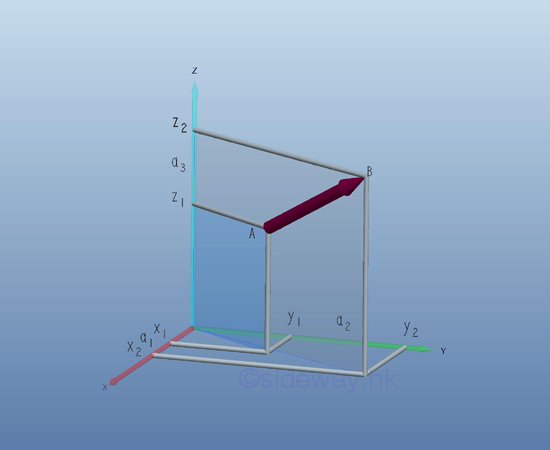Since a point can be considered as a geometric object in a three dimensional space, a point can be represented by the coordinates of a coordinate system such that a vector can be expressed algebraically. The most common system for a three dimensional space is the rectangular coordinate system called Cartesian coordinate system with three mutually perpendicular straight axes of same scale. A vector 𝒂 with initial point 𝐴 and terminal point 𝐵 can be expressed in term of coordinates 𝐴(𝑥1,𝑦1,𝑧1) and 𝐵(𝑥2,𝑦2,𝑧2). The vector 𝒂 can also be interpreted as a displacement vector 𝐴𝐵 displaced from point 𝐴 to point 𝐵. And the geometry of the directed line segment corresponding to the displacement vector can be specified by the numbers 𝑎1=𝑥2-𝑥1, 𝑎2=𝑦2-𝑦1, and 𝑎3=𝑧2-𝑧1 with respect to point 𝐴. These numbers are called components of the vector 𝒂 with respect to the corresponding Cartesian coordinate system because vector 𝒂 can be represented by these components. That is `𝒂=𝐴𝐵=(𝑥2-𝑥1,𝑦2-𝑦1,𝑧2-𝑧1)=(𝑎1,𝑎2,𝑎3)` And the length or the magnitude |𝒂| of vector 𝒂 can be determined by the Pythagorean theorem geometrically.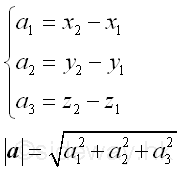As the components 𝑎1, 𝑎2, and 𝑎3 of vector 𝒂 are derived from the end points of the vector by subtracting the coordinates of initial point from the coordinates of the terminal point, the components of vector are independent of the choice of the initial point of the vector and are dependent on the magnitude and direction of the vector only. In other words, the components of vector 𝒂 is a free vector bounded to point 𝐴 with respect to the corresponding Cartesian coordinate system.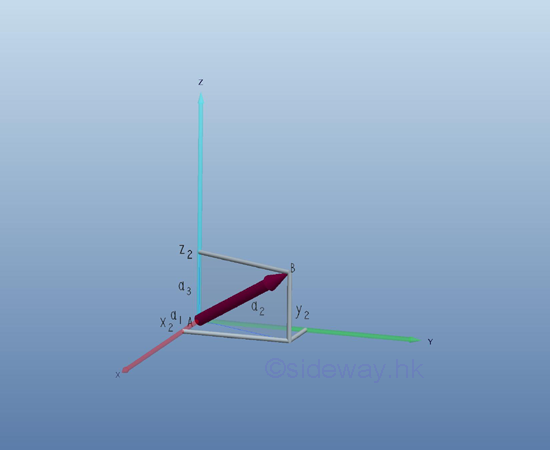Physically, a free vector can be translated arbitrarily to indicate a vector quantity at a point having equal vector quantity. The terminal point of the vector can be determined uniquely once the initial point of the vector is fixed. If the initial point of a vector is located at the origin, the components of the vector are then equal to the coordinate of the terminal point with respect to the corresponding Cartesian coordinate system. The vector with initial point bounded at the origin is called position vector. Therefore, any vector in three dimensional space can be represented by a position vector by translating the initial point of the vector to the origin. And for a specified Cartesian coordinate system, each vector in space can be mapped to ordered triple components in one-to-one relation with respect to the corresponding Cartesian coordinate system. In other words, two vectors 𝒂 and 𝒃 are equal if and only if the corresponding component of two vectors are equal. That is 𝑎1=𝑏1, 𝑎2=𝑏2, and 𝑎3=𝑏3.
As both the magnitude and the direction of the directed line segment of the corresponding vector can be obtained from the components of the corresponding vector, the geometry of the directed line segment in three dimensional space can also be determined by the ordered triple components of the vector in one-to-one relation with respect to the corresponding Cartesian coordinate system.
However, the vector representation of quantity with magnitude and direction is always dependent on the choice of coordinate system.

ID: 191201202 Last Updated: 12/12/2019 Revision: 0 Ref:References

1. Robert C. Wrede, 2013, Introduction to Vector and Tensor Analysis
2. Daniel Fleisch, 2012, A Student’s Guide to Vectors and Tensors
3. Howard Anton, Chris Rorres, 2010, Elementary Linear Algebra: Applications VersionHome 5

Management

HBR 3

Information

Recreation

Culture

Chinese 1097

English 337

Computer

Hardware 149

Software

Application 198

Digitization 117

Numeric 19

Programming

Web 283

Unicode 494

HTML 65

CSS 58

ASP.NET 92

OS 389

Python 19

Knowledge

Mathematics

Algebra 25

Geometry 18

Calculus 67

Engineering

Mechanical

Rigid Bodies

Statics 92

Dynamics 37

Control

Physics

Electric 27